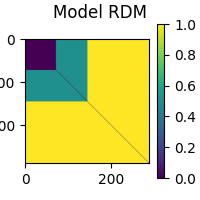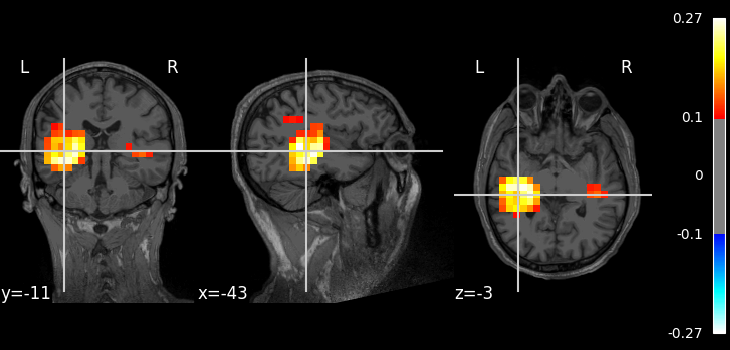# Source-level RSA using a searchlight on volumetric data¶

This example demonstrates how to perform representational similarity analysis (RSA) on volumetric source localized MEG data, using a searchlight approach.

In the searchlight approach, representational similarity is computed between the model and searchlight “patches”. A patch is defined by a seed voxel in the source space and all voxels within a given radius. By default, patches are created using each voxel as a seed point, so you can think of it as a “searchlight” that scans through the brain.

The radius of a searchlight can be defined in space, in time, or both. In this example, our searchlight will have a spatial radius of 1 cm. To save computation time, we will only perform the RSA on a single time point, but feel free to experiment with specifying a temporal radius and performing the RSA in time as well.

The dataset will be the MNE-sample dataset: a collection of 288 epochs in which the participant was presented with an auditory beep or visual stimulus to either the left or right ear or visual field.

```# sphinx_gallery_thumbnail_number=2

# Import required packages
import mne
import mne_rsa
from nilearn.plotting import plot_stat_map

mne.set_log_level(False)  # Be less verbose
```

We’ll be using the data from the MNE-sample set.

```sample_root = mne.datasets.sample.data_path(verbose=True)
sample_path = sample_root / 'MEG' / 'sample'
mri_dir = sample_root / 'subjects' / 'sample'
```

Creating epochs from the continuous (raw) data. We downsample to 100 Hz to speed up the RSA computations later on.

```raw = mne.io.read_raw_fif(sample_path / 'sample_audvis_filt-0-40_raw.fif')
event_id = {'audio/left': 1,
'audio/right': 2,
'visual/left': 3,
'visual/right': 4}
epochs = mne.Epochs(raw, events, event_id, preload=True)
```

It’s important that the model DSM and the epochs are in the same order, so that each row in the model DSM will correspond to an epoch. The model DSM will be easier to interpret visually if the data is ordered such that all epochs belonging to the same experimental condition are right next to each-other, so patterns jump out. This can be achieved by first splitting the epochs by experimental condition and then concatenating them together again.

```epoch_splits = [epochs[cl] for cl in ['audio/left', 'audio/right',
'visual/left', 'visual/right']]
epochs = mne.concatenate_epochs(epoch_splits)
```

Now that the epochs are in the proper order, we can create a DSM based on the experimental conditions. This type of DSM is referred to as a “sensitivity DSM”. Let’s create a sensitivity DSM that will pick up the left auditory response when RSA-ed against the MEG data. Since we want to capture areas where left beeps generate a large signal, we specify that left beeps should be similar to other left beeps. Since we do not want areas where visual stimuli generate a large signal, we specify that beeps must be different from visual stimuli. Furthermore, since in areas where visual stimuli generate only a small signal, random noise will dominate, we also specify that visual stimuli are different from other visual stimuli. Finally left and right auditory beeps will be somewhat similar.

```def sensitivity_metric(event_id_1, event_id_2):
"""Determine similarity between two epochs, given their event ids."""
if event_id_1 == 1 and event_id_2 == 1:
return 0  # Completely similar
if event_id_1 == 2 and event_id_2 == 2:
return 0.5  # Somewhat similar
elif event_id_1 == 1 and event_id_2 == 2:
return 0.5  # Somewhat similar
elif event_id_1 == 2 and event_id_1 == 1:
return 0.5  # Somewhat similar
else:
return 1  # Not similar at all

model_dsm = mne_rsa.compute_dsm(epochs.events[:, 2], metric=sensitivity_metric)
mne_rsa.plot_dsms(model_dsm, title='Model DSM')
``````<Figure size 200x200 with 2 Axes>
```

This example is going to be on source-level, so let’s load the inverse operator and apply it to obtain a volumetric source estimate for each epoch.

```inv = mne.minimum_norm.read_inverse_operator(
sample_path / 'sample_audvis-meg-vol-7-meg-inv.fif')
epochs_stc = mne.minimum_norm.apply_inverse_epochs(epochs, inv, lambda2=0.1111)
```

Performing the RSA. This will take some time. Consider increasing `n_jobs` to parallelize the computation across multiple CPUs.

```rsa_vals = mne_rsa.rsa_stcs(
epochs_stc,                    # The source localized epochs
model_dsm,                     # The model DSM we constructed above
src=inv['src'],                # The inverse operator has our source space
stc_dsm_metric='correlation',  # Metric to compute the MEG DSMs
rsa_metric='kendall-tau-a',    # Metric to compare model and EEG DSMs
temporal_radius=None,          # Don't perform search light over time
tmin=0.09, tmax=0.11,          # Time interval to analyze
n_jobs=1,                      # Only use one CPU core. Increase this for more speed.
verbose=False)                 # Set to True to display a progress bar
```

Here is how to plot the result using nilearn.

```img = rsa_vals.as_volume(inv['src'], mri_resolution=False)
t1_fname = mri_dir / 'mri' / 'T1.mgz'
plot_stat_map(img, t1_fname, threshold=0.1)
``````<nilearn.plotting.displays._slicers.OrthoSlicer object at 0x0000026781621370>
```

Total running time of the script: ( 0 minutes 35.769 seconds)

Gallery generated by Sphinx-Gallery